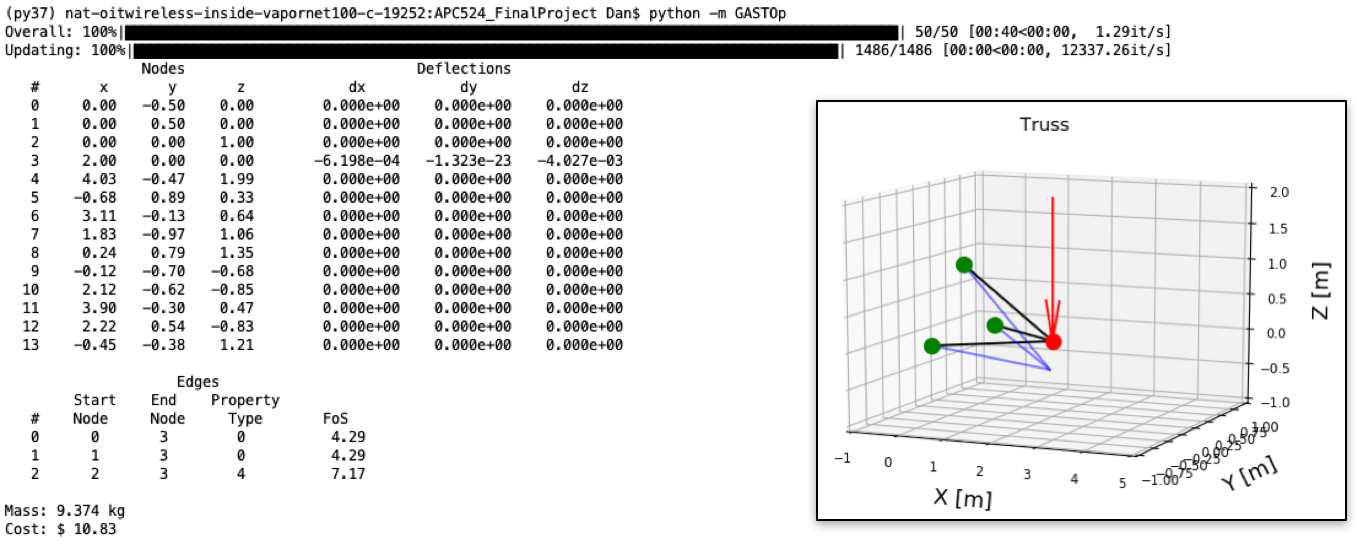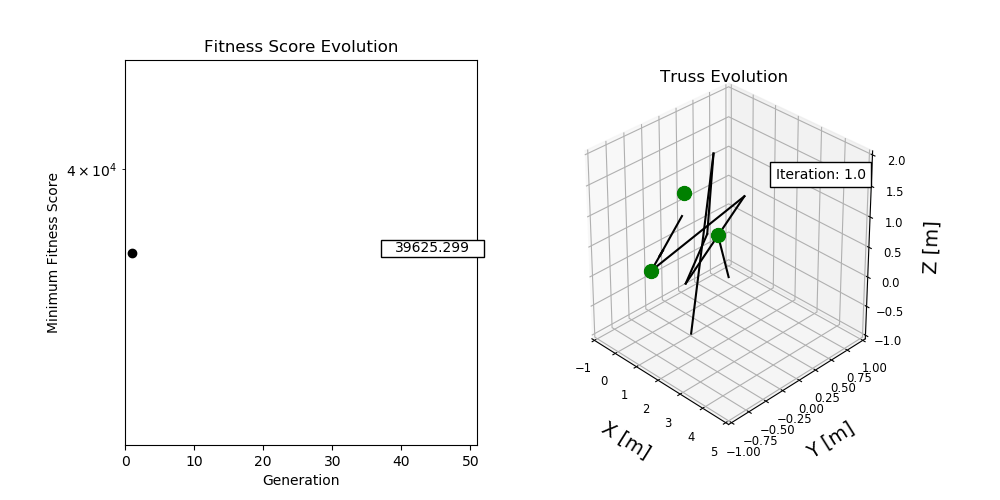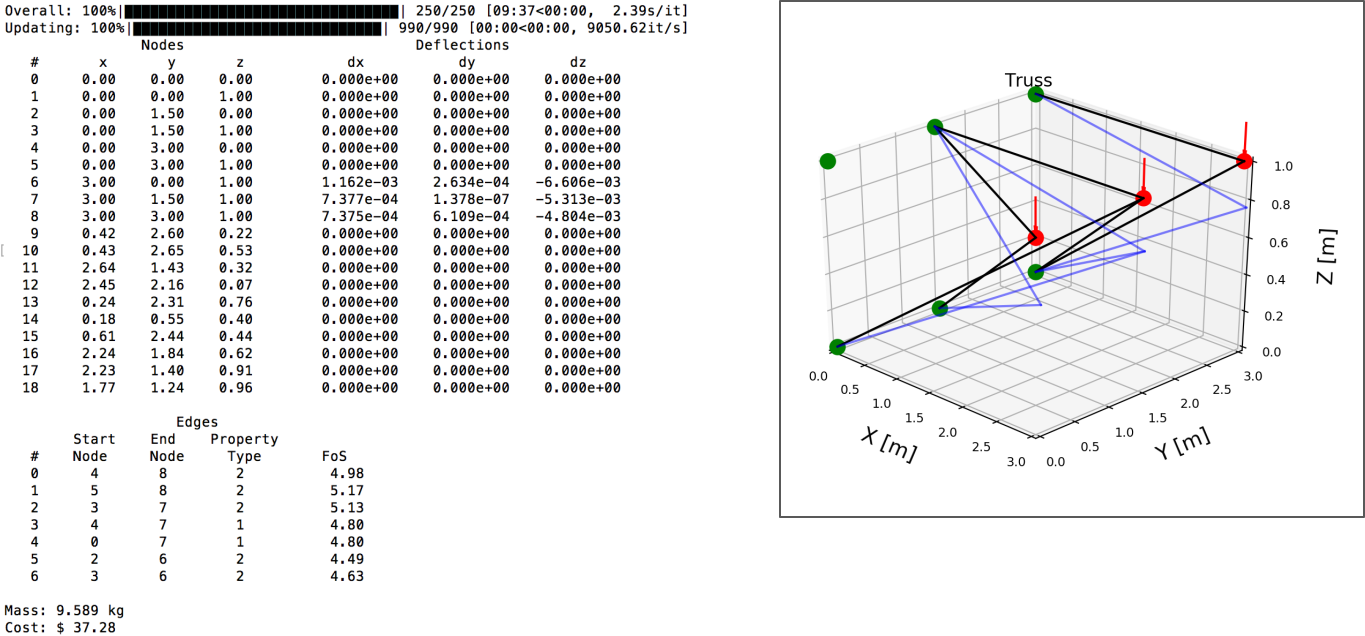# Examples¶

## Pyramid Example Configuration File¶

[general]
user_spec_nodes = '[[0,-.5,0],[0,.5,0],[0,0,1],[2,0,0]]'
fixtures = '[[1,1,1,1,1,1],[1,1,1,1,1,1],[1,1,1,1,1,1],[0,0,0,0,0,0]]'
num_rand_nodes = 10 # int
num_rand_edges = 10 # int
properties_path = 'gastop-config/properties.csv'
domain = '[[-1, -1, -1], [5, 1, 2]]'

[fitness_params]
equation = weighted_sum
[[parameters]]
goal_fos = 4
critical_nodes = ''
w_fos = 10000
w_mass = 1
w_deflection = 100

[evaluator_params]
struct_solver = mat_struct_analysis_DSM
mass_solver = mass_basic
interferences_solver = blank_test
cost_solver = cost_calc

[ga_params]
pop_size = 1500
num_generations = 50
num_elite = 15
percent_mutation =
percent_crossover =
save_frequency = 5
save_filename_prefix = Recorded_States_

[monitor_params]
progress_fitness = True
progress_truss = True

# optional stuff

[random_params]
rng_seed =

[crossover_params]
node_crossover_method =
edge_crossover_method =
property_crossover_method =
[[node_crossover_params]]
[[edge_crossover_params]]
[[property_crossover_params]]

[mutator_params]
node_mutator_method =
edge_mutator_method =
property_mutator_method =
[[node_mutator_params]]
std =
[[edge_mutator_params]]
proportions =
[[property_mutator_params]]
proportions =

[selector_params]
method =
[[method_params]]
tourn_size =
tourn_prob =


## Pyramid Example Results¶

For this example there are three nodes that are fixed in all six degrees of freedom at XYZ locations [0,-.5,0] , [0,.5,0] , [0,0,1]. A load is applied at [2,0,0] in the negative Z direction with a magnitude of 10,000 N. The genetic algorithm uses a population size of 1500, runs for 50 generations, and is the structure is required to have a safety factor of four. The displacement is minimized for node 3 which is the loaded node at [2,0,0]. To run this example the command is as shown below:

$gastop gastop-config/struct_making_test_init2.txt  The results of this simulation are:Here the fixed nodes are shown in green, the loads are shown in red, and the truss is shown in black. The blue lines show how the truss deforms under the load magnified by a factor of 50 to be visible. The evolution of the optimization algorithm is shown here:## Cantilever Example Results¶ For this example there are six nodes that are fixed in all six degrees of freedom at XYZ locations [0,0,0], [0,0.001,1], [0,1.5,0], [0,1.5001,1], [0,3,0], [0,3.0001,1]. Three loads are applied at [3,0,1], [3,1.5,1], [3,3,1] in the negative Z direction, each with a magnitude of 1000 N. The genetic algorithm uses a population size of 1000, runs for 250 generations, and is the structure is required to have a safety factor of four. The displacement is minimized for node 7 which is the middle loaded node at [3,1.5,1]. To run this example the command is as shown below: $ gastop gastop-config/init_cantilevered_test.txt


The results of this simulation are:Here the fixed nodes are shown in green, the loads are shown in red, and the truss is shown in black. The blue lines show how the truss deforms under the load magnified by a factor of 50 to be visible.